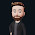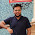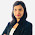VHDL coding tips and tricks: Digital clock in VHDL

## Monday, March 15, 2010

### Digital clock in VHDL

Here is a program for Digital clock in VHDL.The module has one input 'clk' and 3 outputs.Each output represents time in seconds,minutes and in hours.The module has two processes.One of them generate the necessary clock frequency needed to drive the digital clock.The main clock frequency applied to the module is 100 MHz.But our digital clock has to be driven at only 1 Hz.The first process does the necessary clock division needed for this.
The second process increment the seconds,minutes and hours etc when the conditions are met.For example at every clock cycle we increment 'seconds'.Whenever seconds reaches the value '60' we increment 'minutes' by 1.Similarly whenever minutes reach '60' we increment 'hours' by 1.Once hours reaches the value '23' we reset the digital clock.The VHDL code for digital clock is given below:

library ieee;
use ieee.std_logic_1164.all;
use ieee.std_logic_arith.all;
use ieee.std_logic_unsigned.all;

entity digi_clk is
port (clk1 : in std_logic;
seconds : out std_logic_vector(5 downto 0);
minutes : out std_logic_vector(5 downto 0);
hours : out std_logic_vector(4 downto 0)
);
end digi_clk;

architecture Behavioral of digi_clk is
signal sec,min,hour : integer range 0 to 60 :=0;
signal count : integer :=1;
signal clk : std_logic :='0';
begin
seconds <= conv_std_logic_vector(sec,6);
minutes <= conv_std_logic_vector(min,6);
hours <= conv_std_logic_vector(hour,5);

--clk generation.For 100 MHz clock this generates 1 Hz clock.
process(clk1)
begin
if(clk1'event and clk1='1') then
count <=count+1;
if(count = 50000000) then
clk <= not clk;
count <=1;
end if;
end if;
end process;

process(clk)   --period of clk is 1 second.
begin

if(clk'event and clk='1') then
sec <= sec+ 1;
if(sec = 59) then
sec<=0;
min <= min + 1;
if(min = 59) then
hour <= hour + 1;
min <= 0;
if(hour = 23) then
hour <= 0;
end if;
end if;
end if;
end if;

end process;

end Behavioral;
This digital clock can be used as a component in your main program.If you want to display the time in LCD panel or use a speaker to tell the time then you need to write the appropriate VHDL code for interfacing with such components in your FPGA board.Such programs may vary depending upon the board and FPGA chip you are using.

#### 34 comments:

1.Nice code... But I only want to ask one ques, say i have to set clock manually. How will that can be done..

2.awsome! but how will it look like if i want the results to be displayed on the LCD

3.i still don't understand how to generate the necessary clock frequency. For 100 MHz clock, why you wait for 'count' till 50000000 instead of 100000000?

1.we are counting for half cycle and changing the value to the high state again

4.@timmy : look at the code snippet carefully.What we are doing here is that a signal 'clk' is toggled whenever the count reaches 50m(50 million). This means that
clk='1' for 50m clock cycles of clk1.
then clk='1' for 50m clock cycles of clk1.and this toggling goes on.
In effect, one clock cycle of clk = 100m clock cycles of clk1.

I hope now you understand.

5.can u postt the testbench for it?

6.hey....how can we adjust the time of this clock?? i mean if we press the BTN1 it should increment or decrement the minutes.....do you have a sample code for this..??

7.Hey...do you ppl have a code which can adjust the time of the clock using its different buttons or switches??i mean if switch/button is pressed it increment or decrements the time??kindly help out

8.@Uzar : This is the basic code for the clock. I dont have the code for the time setting part.

9.hi i wnt vhdl code for interfacing gsm and gpsm modem to fpga

10.can u send me another code for digital clock

11.hi can u send me another code for digital clock

12.how to set mode pin in clock using vhdl code?

13.can anyone help to write a vhdl program for analog to digital converter

14.Hi. Sorry for this newbie question. Why do you use integer type for sec,min,hour and then convert to std_logic_vector? Why aren´t sec,min,hour declared as std_logic_vector in the beginning?
Regards,
Maia

15.what value i am supposed to give as/ for "clk1"...?? i mean what is input here precisely if i want to see simulation wave-forms for tis program?? ...reply ASAP....im in so hurry....plz....

16.hey what as an input i am supposed to put here in "clk1"???...reply ASAP....i want to see simulation waveforms in xilinx and model sim also...plz reply ASAP........

17.please post code for a Stop Watch, which displays the time in three decimal digits, and counts from 00.0 to 99.9 seconds and wraps around. It contains a synchronous clear signal, clr, which
returns the count to 00.0, and an enable signal, go, which enables and suspends the
counting. This design is basically a BCD (binary-coded decimal) counter, which counts
in BCD format. Our Broad has a 100-MHz clock;

18.Your code doesn't work. The time increments from 22:58:59 to 00:00:00 due to crappy coding.

19.What should be input parameters while creating testbench? What should be clock high and low time,input setup time,output valid delay,offset,initial length of testbench in nanoseconds????
plz reply asap...coz m not getng op waveform

20.how will it look like if i want the results to be displayed on the LCD??

1.for that u have to create UCF file for display.and need to refresh the LCD pannel at very low time like 50msec such that it has to keep on updating.

21.Hey...just for curiosity...I wanted to extend the concept to show date,month and year also....but actly finding some problem with month identification...can anyone help????

22.hi
why in vhdl i need to write
if sec = 59 then sec <= 0
while in verilog, to get same result, i need to write
if (sec == 60) begin sec = 0 end
can you give explanation?

1.hi!
sec, min and hour were declared as signals and not variables. A signal will take a full clock cycle to change and so, the value zero will be passed to sec in the following clock circle where the value was actually supposed to be 60.

23.Hey anyone know how can we record time ? Does anyone have code for that ?

24.Hiii.....plz tell me how to burn this code on fpga

25.hello, i want to design a circuit in which 2 counters will count simultaneously such that first counter will count from 0 to 7, after that second counter will count 1, again first counter will count 0 to 7 and second counter will count 2.

1.You can do it in a very similar way as in this digital clock code. try to understand how this code works and I am sure you can do it yourself. I also help students for a fee in their projects.

26.Hello, I've some troubles with this code, can U help me pls?

Parsing architecture of entity .
ERROR:HDLCompiler:841 - "C:\Users\Tom\Desktop\VSB\1. semestr\PHP\projekty\Clock_project\clk.vhd" Line 46: Expecting type std_logic for .
ERROR:HDLCompiler:841 - "C:\Users\Tom\Desktop\VSB\1. semestr\PHP\projekty\Clock_project\clk.vhd" Line 47: Expecting type std_logic for .
ERROR:HDLCompiler:841 - "C:\Users\Tom\Desktop\VSB\1. semestr\PHP\projekty\Clock_project\clk.vhd" Line 48: Expecting type std_logic for .
ERROR:HDLCompiler:854 - "C:\Users\Tom\Desktop\VSB\1. semestr\PHP\projekty\Clock_project\clk.vhd" Line 41: Unit ignored due to previous errors.
VHDL file C:\Users\Tom\Desktop\VSB\1. semestr\PHP\projekty\Clock_project\clk.vhd ignored due to errors

27.This comment has been removed by the author.

28.Hi, can some one send me the block diagram of digital clock. like how can we make it to display it on VGA. I have VGA code and clock code but i dont know how to combine them together.

29.Can you write a code to connect LED Xilinx board , please answer me as soon as you can .thank you

30.Hello please can you send test file ??